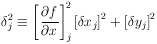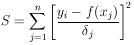### APPENDIX III. LEAST SQUARES WITH ERRORS IN BOTH VARIABLES

Experiments in physics designed to determine parameters in the functional relationship between quantities x and y involve a series of measurements of x and the corresponding y. In many cases not only are there measurement errorsyi for each yj, but also measurement errorsxj for each xj. Most physicists treat the problem as if all thexj = 0 using the standard least squares method. Such a procedure loses accuracy in the determination of the unknown parameters contained in the function y = f (x) and it gives estimates of errors which are smaller than the true errors.

The standard least squares method of Section 15 should be used only when all thexj <<yi. Otherwise one must replace the weighting factors 1 /i2 in Eq. (24) with (j)-2 where(36)

Eq. (24) then becomes(37)

A proof is given in Ref. 7.

We see that the standard least squares computer programs may still be used. In the case where y =1 +2x one may use what are called linear regression programs, and where y is a polynomial in x one may use multiple polynomial regression programs. The usual procedure is to guess starting values for ðf / ð x and then solve for the parametersj* using Eq. (30) withj replaced byj. Then new [ðf / ð x]j can be evaluated and the procedure repeated. Usually only two iterations are necessary. The effective variance method is exact in the limit that ðf / ð x is constant over the regionxj. This means it is always exact for linear regressions.